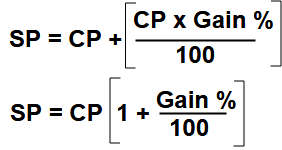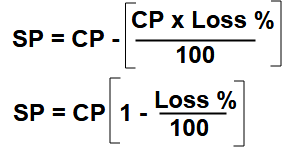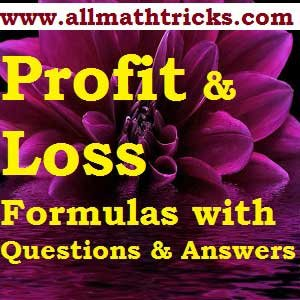# Profit and Loss Formulas with Questions and Answers | Quantitative Math

## Formulas for Profit and loss and practice sums | Exercise – 1

The terminology used in profit and loss chapter is

Cost Price (CP)

Selling price (SP)

Profit (P),  Loss(L), Profit percent, Loss percent, Gain , Gain percent

Variable cost, Fixed cost,

Mark Up, Marked price,

Discounts, Successive discounts,

Break even point,

Faulty balances,  faulty measure , Chain rules … etc

Now we see one by one definitions, its related formulas with example off profit and loss sums.

Cost Price ( CP ) : The price at which a person buys a product is the cost price of the product for that person. The cost price can be also defined as the amount paid or expended in either purchasing or producing an article.

Selling price (SP) : The Price at which a person sells a product is the sales price of the product for that person. It can be also defined as the amount got when an product is sold is called as the selling price (SP).

Profit or gain : When a person able to sell a article at a price higher then its cost price, then we say that he got profit or gain.

i.e The selling price is more than the cost price then called profit or gain. (CP < SP )

Loss : When a person able to sell a article at a price lower then its cost price, then we say that he incurred loss.

i.e The selling price is lower than the cost price then called loss. (CP > SP )

Impotent Note : Th percentage of profit or loss is always reckoned on cost price (CP) unless otherwise stated.

Formulas on Profit and Loss :

Profit (Gain ) =  SP – CP  ( i.e SP = Profit + CP )

Loss =  CP – SP ( i.e CP = Loss + SP )

Profit Percentage = [ Profit x  100] / CP

Profit = [ profit% x CP ] / 100

Loss Percentage =  [ Loss x  100] / CP

Loss = [ Loss % x CP ] / 100

Sell Price = CP + Gain or ProfitSell Price = CP – LossThe profit or gain can be represented by the amount of items or good or articles left. Hence profit when money is equated is given by items left and the cost in this case is represented by items sold.

The Profit percent =[  Articles left / Articles Sold ] x 100.

Practice Sums related to above profit and loss formulas

Example -1 : A person purchased an article for  ‎₹ 150. If he sells it at a 20% profit then find his selling price.

Solution : Formula : –  SP = CP [ 1 +  ( Gain % x 100) ]

SP = 150 [ 1 + (20/100) ]

= 150 x 1.2 = 180.

The article selling price is ₹ 180.

Example – 2 : By selling a watch for ₹ 990, a shopkeeper incurs a loss of 10%. Find the cost of the watch for shopkeeper.

Solution : Formula : –  SP = CP x  [ 1 –  ( Loss % x 100) ]

990 = CP x [ 1 – (10/100) ]

CP = 990 / 0.9 = 9900 / 9 = ₹ 1100.

Example – 3 : By selling a product for ‎₹ 720, a loss of 10% is obtained. At what price the product must be sold to get 20% gain?

Solution :

1.Formula for first case

SP = CP + [ 1 –  ( %Loss x 100) ]

720 = CP + ( 1 – 0.1 )

CP = 720 / 0.9 = ₹800.

2. Formula for second  case

SP = CP  [ 1 +  ( Gain % x 100) ]

SP = 800  [ 1 + (20/100) ]

= 800 x 1.2 = ₹ 960.

I.e The seller sold the product at ₹ 960 then he will gain 20%.

Example – 4 : A person sold an article at 12% profit. Had he sold it for ₹ 18. more he would have gained 18%. What is the cost price of the article?

Answer: Formula :-  SP = CP  [ 1 +  ( Gain % x 100) ]

In first case

SP = CP ( 1 + 0.12) = 1.12 CP

In second case

SP = CP ( 1 + 0.18) = 1.18 CP

So the difference between first and second case is ₹ 18.

i.e 1.18CP – 1.12CP = 18.

CP = 18 / 0.06 = 1800 / 6 = 300.

The article cost price is ₹ 300.

Example – 5 : A manufacturer  sold a product for ₹ 2400 and made a profit of 25% in the process. Find his profit percent if he had sold his goods for ₹2040.

Answer : formula – SP = CP  [ 1 +  ( Gain % x 100) ]

In first case SP = 2400

2400 = CP  [1 + (25/100) ]

CP = 2400 / 1.25 = 240000 / 125 = 1920.

In second case SP = 2040.

Then Profit = 2040 – 1920 = 120.

Profit % = [ Profit x  100] / CP

= 120 x 100 / 1920 = 6.25 %.

Example – 6  : Tom sells a bicycle to Ram at 25% profit, Ram sells it to Hari at 20% loss. If Hari pays ₹1500 for it, at what price Tom purchase it?

First find the Ram cost price  and Ram sold price  ₹ 1500

Formula :  SP = CP  [ 1 –  ( %Loss x 100) ]

1500 = CP [ 1 – (20 / 100) ]

CP = 1500 / 0.8  = 15000/ 8  = 1875.

Here Ram cost price equal to Tom sell price.

In second case Tom got 20% profit and his sell price ₹ 1875.

SP = CP  [ 1 +  ( Gain % x 100) ]

1875 = CP [ 1 + (25/100) ]

CP = 1875  / 1.20 = 187500 / 125 = 1500.

Example – 7 : A fruit vendor recovers the cost of 25 mangoes by selling 20 mangoes. Find his profit percent.

Answer: Formula – The Profit percent = [  mangoes left / mangoes Sold ] x 100.

Profit % = [ ( 25 -20) / 20 ] x 100 = 25%

The fruit vendor profit is 25%.

Example – 8 : If the cost of 20 pens is equal to the selling price of 16 pens. what is the gain or loss%

Answer:Here The cost pens less than the selling pens, so in this process get profit

Formula :- The Profit percent = [  pens left / pens Sold ] x 100.

Profit % = [ ( 20 -16) / 16 ] x 100 = 400 / 16 = 25%

The profit is 25%.

Example – 9 : While selling of 5 pens, A person get profit equal to selling of 2 pens. Find the profit percent.

Answer : Write the profit equation.

Selling Price =  Profit + Cost Price

5 SP = 2SP + 5CP

3 SP = 5CP

SP / CP  = 5 / 3

The Profit percent = [  pens left / pens Sold ] x 100.

Profit % = ( 2 / 3 ) x 100 = 200 / 3 = 66.66%.

Example – 10 : While selling of 5 pens, A person incurred loss equal to sold of 3 pens. Find the loss percent.

Answer : Write the loss equation.

Selling Price = Cost Price – Loss

5 SP = 5CP – 3SP

8 SP = 5CP

SP / CP  = 5 / 8

Loss % = ( 3/ 8 ) x 100 = 300 / 8 = 37.5%.

Example – 11 : While selling of 6 pens, A person get profit equal to cost of 2 pens. Find the profit percent.

Answer : Write the profit equation.

Selling Price =  Profit + Cost Price

6 SP = 2CP + 6CP

6 SP = 8 CP

The Profit percent = [  pens left / pens Sold ] x 100.

Profit % = ( 2 / 6 ) x 100 = 200 / 6 = 33.33%

Example – 12 : While selling of 10 pens, A person incurred loss equal to cost price of 3 pens. Find the loss percent.

Answer : Write the loss equation.

Selling Price = Cost Price – Loss

10 SP = 10CP – 3CP

10 SP = 7 CP

SP / CP  = 7 / 10

Loss % = ( 3/ 10 ) x 100 = 300 / 10 = 30%.For more exercises on Profit and Loss chapter please go through the below links

Exercise – 2

Exercise – 3

Exercise – 4

Some related Topics in Quantitative aptitude

The Concepts of number system the mathematics

Divisibility Rules of numbers from 1 to 20 | Basic math education

Simple interest and Compound interest formulas with examples

Percentage formulas | percentage calculations with examples

Circle formulas in math | Area, Circumference, Sector, Chord, Arc of Circle

Types of Triangles With examples | Properties of Triangle

## 4 thoughts on “Profit and Loss Formulas with Questions and Answers | Quantitative Math”#### Sher Singh

(November 15, 2018 - 8:27 pm)

Math all topic#### sivaalluri

(November 16, 2018 - 4:44 pm)

We will provide all topics soon#### Mukeshchandra

(June 10, 2019 - 5:59 am)

Its a fantastic duty sir!and also update formulas of physics and chemistry
Thankyou sir.#### sivaalluri

(July 2, 2019 - 2:44 pm)

ok